• WORK
• TOOLS
• TRAINING
• VIEWS
• CONTACT

A non-ribbon Sankey diagram consists of two sets of nodes connected to each other with links. The nodes of each set are placed along vertical lines. Then, curves are drawn to connect related nodes. Simply put, it’s a stripped down version of a Sankey diagram. Compared to its traditional cousin—the Sankey diagram—non-ribbon Sankey diagrams emphasize the connections between the nodes rather than the additional properties of those links. Here is an example from Bloomberg. Let’s build it in Excel.

The logical way to represent this graph is to use a scatterplot. I have two sets of data. The one on the left includes three points while the data set on the right includes 27 points. So lets define the (x,y) of each point assuming the left data set will be located at x = 0 while the right one is located at x = 1. The points on the vertical axis will be equally spaced as per the following set up. The choice of the upper (0.15 and 0.5) and lower (-0.15 and -0.5) bounds is arbitrary. Since we have 27 points on the right side, then the spacing between the points is 1 / 26 = 0.0384615.

Next, lets constrain the vertical axis minimum and maximum bounds to -0.5 and 0.5 respectively and that of the horizontal axis to 0 and 1 respectively. The chart should look like this:

# HOW TO CREATE A NON-RIBBON SANKEY DIAGRAM IN EXCEL

HOW TO CREATE A NON-RIBBON SANKEY DIAGRAM IN EXCEL

Lets do some cleaning. Delete the chart title, the legend, the vertical and horizontal grids and the vertical and horizontal axis. Turn off the border of the chart area and the chart should look like this:

Next, lets change the shape and fill color of the markers. For the set on the right we can use the built-in square shape, but for the set on the left we need to use a picture. To do that, draw a rectangular shape (in my case 0.04" wide and 0.14" high) anywhere on the worksheet using the Shapes in the Illustration Group under the Insert tab. Then, copy the newly created shape to the Clipboard by pressing Ctrl + C. Next, select the markers of the data series on the left and paste the shape by pressing Ctrl + V. The chart should look like this:

Next we need to prepare the setup for the input data matrix of the chart. For that we'll use a matrix made of 27 rows and 3 columns with ones and zeros—that is, one if there is link between the related nodes and zero otherwise. The input matrix is as follows:

Since we have the labels of each series, I can add them to the chart using the Value From Cells option and the chart should look like this:

To fix the problem of the labels overlapping the markers, we can adjust the size of the plot area in a way to clear space on the left and the right for the labels to fit in as below:

Next, I'll use a monospaced font such as Lucida Sans Typewriter to fix the ragged carpet problem of the labels on the right. Equal width font bring order to the chart by unifying the width of the labels as below:

Now that the backbone of the chart is done we're ready to create the links between the nodes. And here comes the interesting part. A warning however, the next steps require some heavy use of mathematics—Bézier curves.

According to Microsoft, by definition: "a Bézier spline is a curve specified by four points: two end points (p0 and p3) and two control points (p1 and p2). The curve begins at p0 and ends at p3. The curve doesn't pass through the control points, but the control points act as magnets, pulling the curve in certain directions and influencing the way the curve bends."

The following illustration shows a cubic Bézier curve along with its endpoints, control points and the equations to generate the curves:

For this graph, I'm going to use a special case of cubic Bézier curve where the end points and control points share similar y coordinates with P1 abscissa at 0.42 and P2 abscissa at 0.58 as follows:

Each cubic Bézier curve will be made of 10 points, hence we need to generate 10 equally spaced (t) as follows (remember t should be between 0 and 1, both included):

Since we have 3 nodes on the left then we need to create a setup so that we end up with three data series so that we can format each series separately. Therefore, we need to stack 27 Bézier curves (some of them may be #N/A) on top of each other with gaps in between to avoid connecting the end points of 2 consecutive curves. Here is the setup with explanations:

Add the Tencent data series to the scatterplot and the chart will look like this:

Add the Alibaba and Ant data series to the chart, add text boxes for the chart title, sources, annotations and Bloomberg's logo. And the chart should look like this:

Next, lets insert a scatterplot chart with the above defined series. The chart should look like this:

Views > Excel charts > How to create a non-ribbon sankey diagram in excel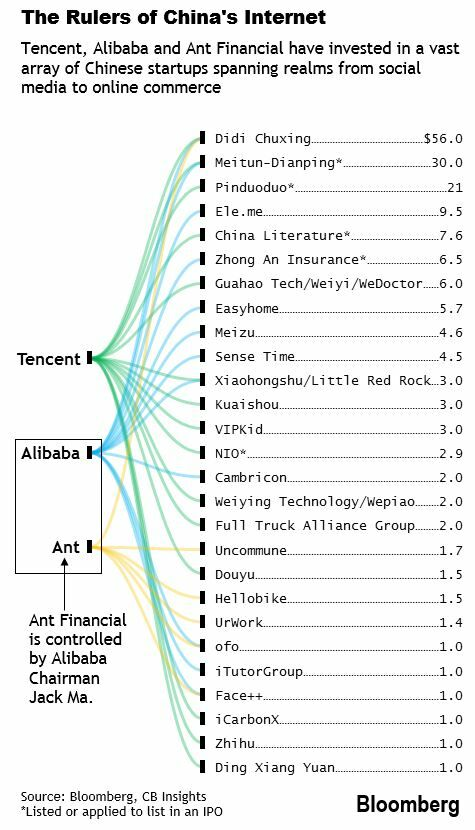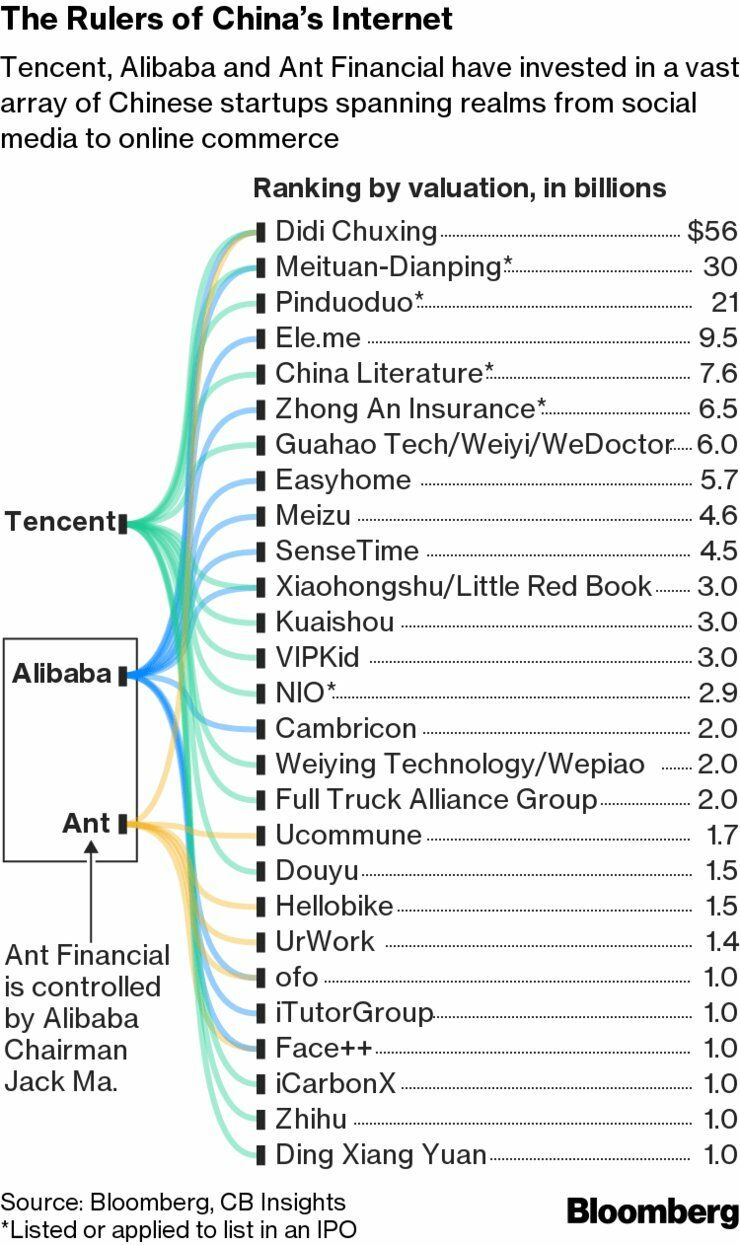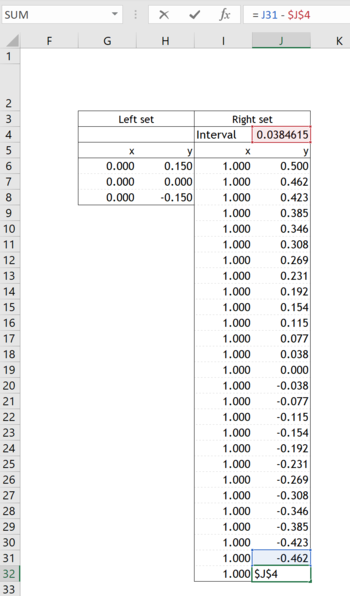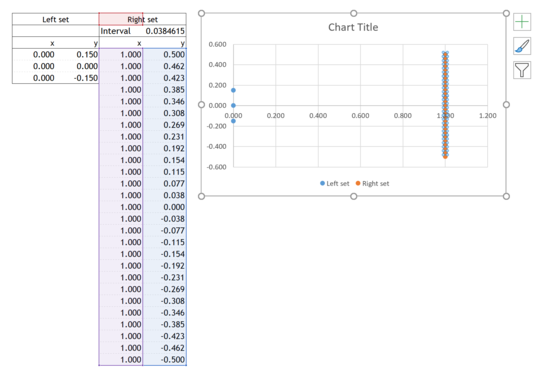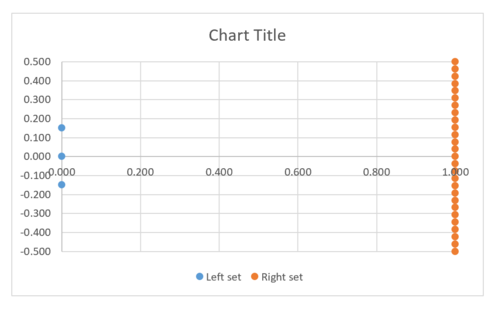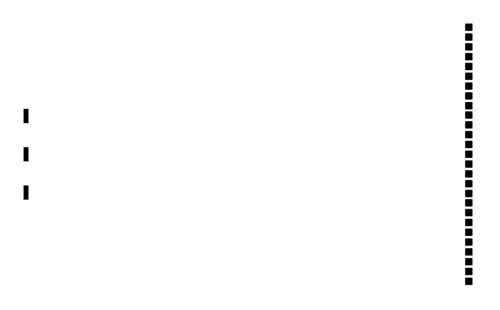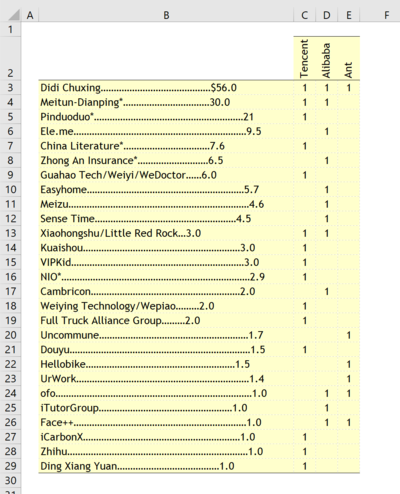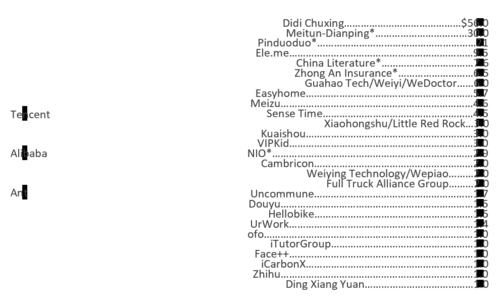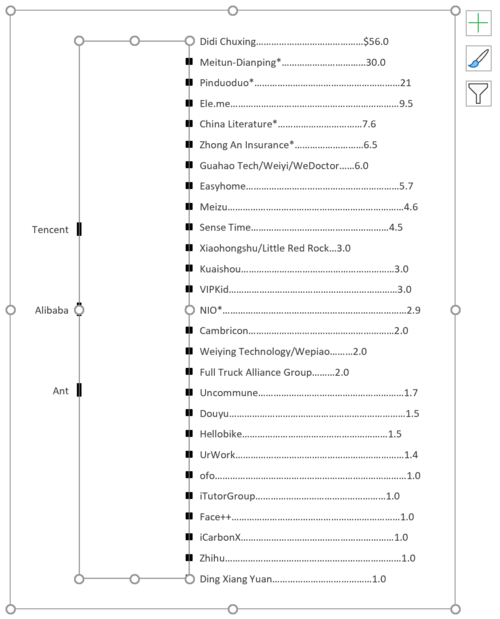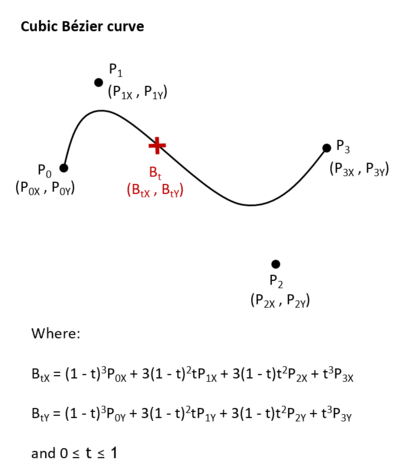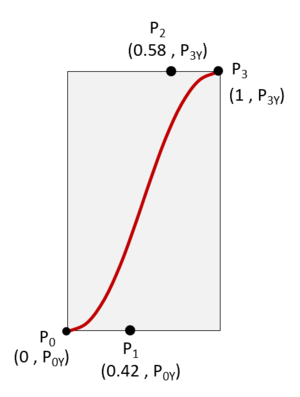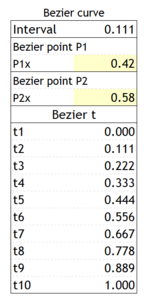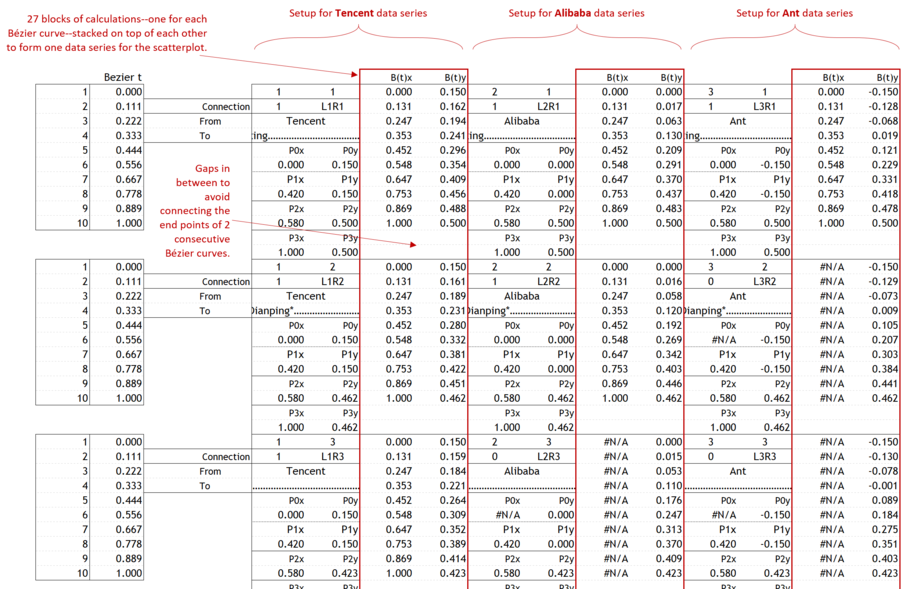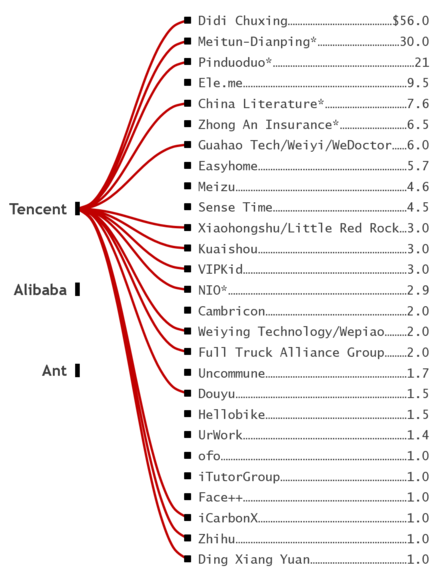You may wonder what would be the impact of the x values P1x =  0.42 and P2x = 0.58 on the shape of the Bézier curves. Lets take a couple of examples.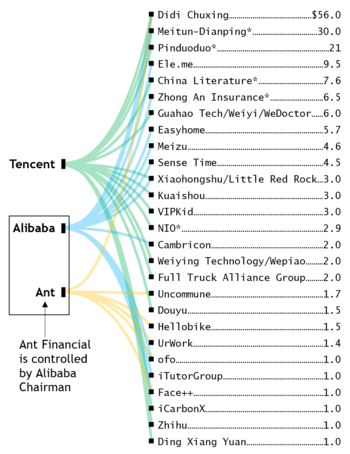P1x =  0.714 and P2x = 0.986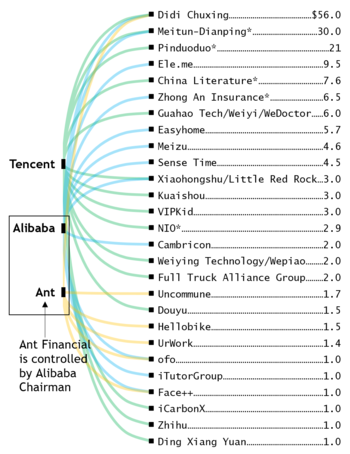P1x =  0.000 and P2x = 0.000

Thank you for reading, and I hope you found this useful. If you have any questions, find me on Twitter and ask me anything.